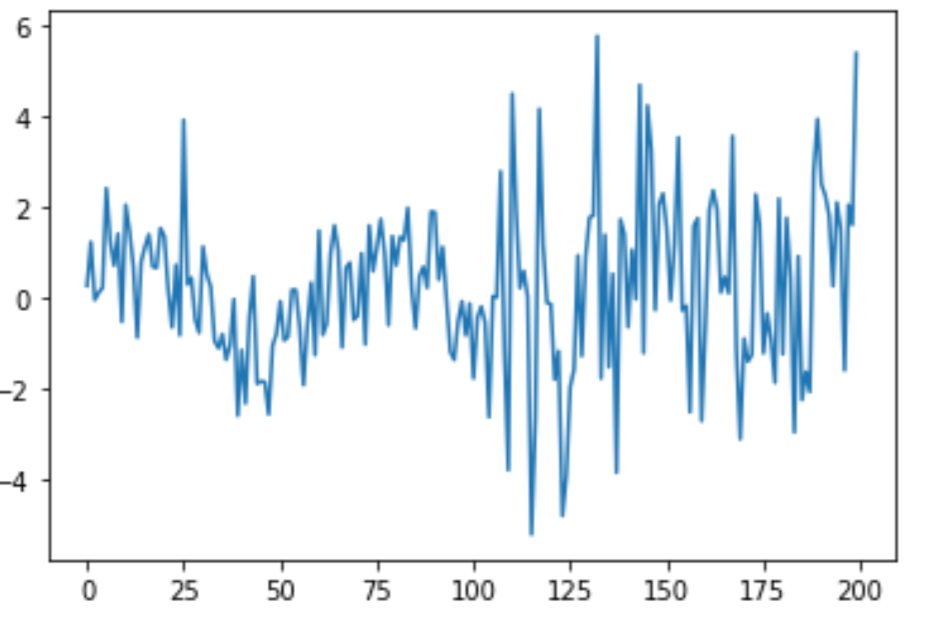Photo by Jeffrey Wegrzyn on Unsplash

# ‘Online’ Kalman Filters for Streaming IoT Data

## Applying Kalman Filters to real-time streaming data

1. Tracking the actual values for highly sensitive sensors
2. Tracking the velocity of objects with uncertainty

# Introduction

The simplest way to understand a Kalman Filter is as follows:

Given a “noisy” signal over time, a kalman filter creates estimates of the true signal.

A deeper explanation:

Given a “noisy” signal, a kalman filter creates an estimate of the true state by parameterizing the signal with the following function:

The current state is defined as `k`. The previous state is `k-1` .

`F` is a state transition model (matrix), applied to the previous state. `B` is a control state model applied to a “control vector,” `u`.

The product of the state transition matrix and the observation at time `k-1` gives an estimate of the state at `k`. Deriving this state transition is outside the scope of this article, however the properties of this matrix and the example provided here can help. Note: there are multiple ways to derive this state transition matrix.

The control state model is only applicable with control inputs, such as the angle of the accelerator pedal in the case of velocity estimates for a vehicle. In these cases, the product of the control state model along with the inputs at time `k-1` also add to the estimate of the true state.

`w` is the noise, assumed to be Gaussian.

Why use Kalman Filters?

Kalman filters are useful because they provide a much better estimate of the true state than other “smoothing” techniques, such as moving averages (exponential and simple). See the example provided below.

# An example of a noisy process

Here, I show a sample ground truth process which evolves over time — a sin wave!

For the first half of my observational window, I add gaussian noise with `mu= 0` and `σ=1`. For the second half, I increase `σ to 2.`

Here’s what this noisy process looks like:Image by Author

For an experiment, I want to test 2 ways of teasing out our ground truth signal.

1. A simple moving average (period=5)
2. A kalman filter

For evaluation, I’ll test the mean squared error of the estimate provided by the predictors listed above against the ground truth (the “un-noised” sin wave)

Here are the MSE results

1. Moving Average: `0.52`
2. Kalman Filter: `0.43`

A nearly 17% reduction in MSE from our baseline!

Here — the kalman estimates are in blue, the moving averages in orange, and the true signal in green. Observe that the kalman filter is more robust to the heteroskedacity in the noise and is overall a better approximation of the true signal.

Because the kalman filter can update it’s internal state with every observation, we can make an online algorithm from it. Here, I add a simple internal window, with a `deque` along with a `maxlen`. Although you can update the internal state with each new observation, I’ve found better empirical results by using a window and updating the state of the filter periodically.

This makes intuitive sense, as the noise of the process can be a latent function that evolves over periodic intervals vs. consistently drifting.

Thanks for reading!

If you liked this, you might like:

Data Scientist